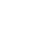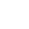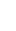HomeModulesArticlesVideosLife EventsCalculatorsQuizJargonExtras

### Investments

 + Investments Overview + Returns from investment + Asset classes + Asset class - cash + Asset class - fixed interest + Asset class - property + Asset class - shares + Tax and investment returns + Diversification and portfolio construction + Relationship between risk and return + Investment risk profiles + Investment methods + Analysis of performance + Performance of managed funds - Performance of investment portfolios + Summary of investments

## Performance of investment portfolios

To calculate a total portfolio return, we will use the returns we have previously calculated, shown in the table.

Let’s assume you bought all of these investments, therefore you have invested a total sum of \$600,000.

There are two ways of calculating the performance; both methods are helpful to know.

The first and most simple calculation of the portfolio return is the total dollar return divided by the dollar investment:

Return = \$26,949 / \$600,000 = 4.49% (0.0449)